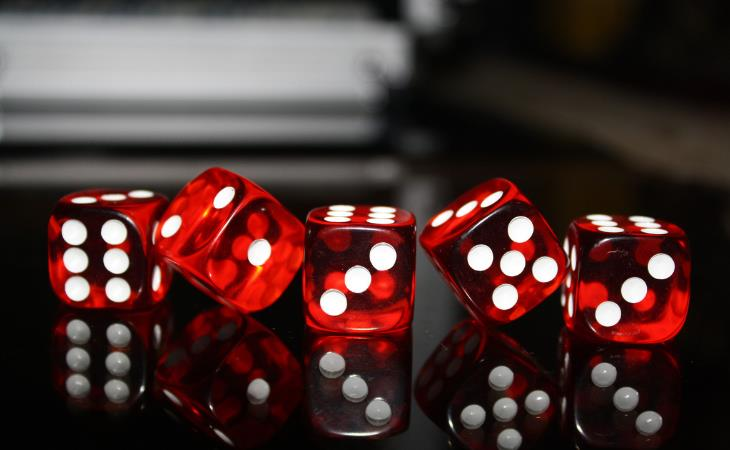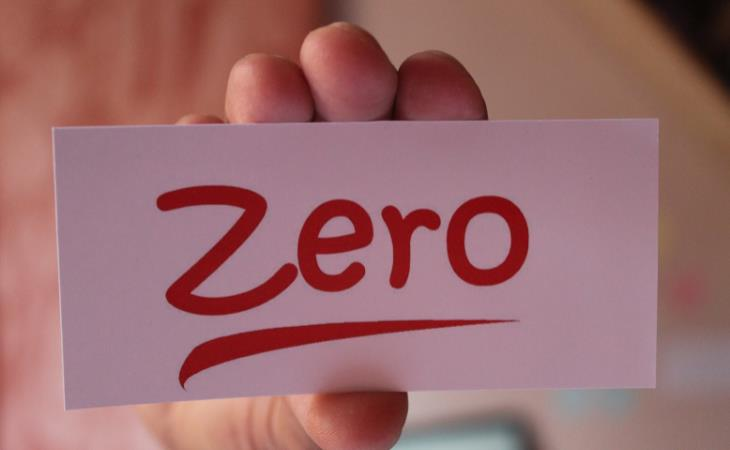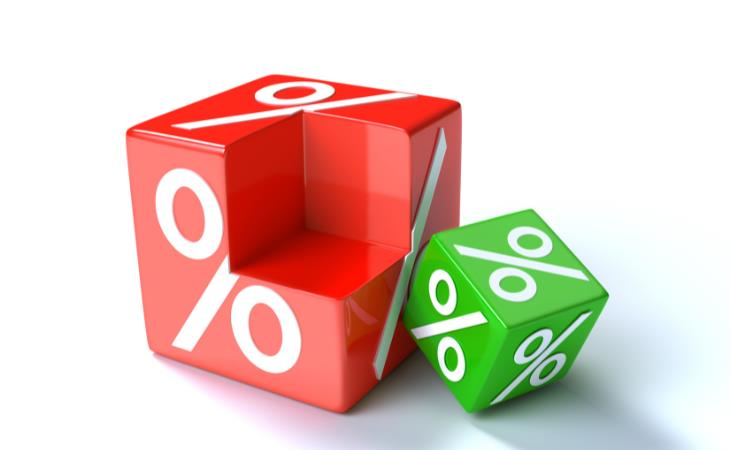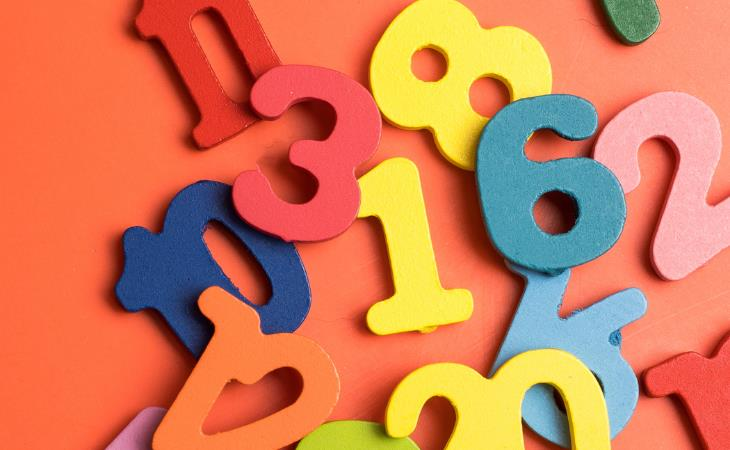# Math Can be Fun- Here Are 13 Cool Facts to Prove it

A+ A-
Markings on animal bones found in archeological excavations prove that math has been around since 30,000 BC. Probably since then to this very day, mathematics has been widely considered a daunting subject for those not gifted with the talent of crunching numbers. It tends to challenge us and make us feel a little bit frustrated when we approach mathematical equations we cannot decipher. In this article, we hope to show you the beauty of math with some light, fun math facts and tips and tricks that may help you in your daily life.

## General fun facts1. The numbers on opposite sides of the dice will always add up to seven.

This rule applies not only to the classic dice but also to any die with any number of faces. The general rule says that opposite sides will add up to the maximum (the highest number on the dice) plus one. On a classic die with six faces, the numbers will add up to seven. The reason for this design is to allow the even distribution of results with each roll of the dice.

2. Once and for all – zero is an even numberA number is even if it is divisible by two or is a multiple of two. Zero is divisible by two: 0:2=0. It is a multiple of two; 0x2=0. Hence, zero is an even number! We can go the other way around and prove that it is not an odd number. An odd number has to be followed by an even number. Zero is followed by one – an odd number – proving, once again, that zero is an even number.

Another interesting fact about zero is that it is not represented in Roman numerals. They used the word "nulla," meaning "nothing," to refer to zero.

3. A simple percentage calculation

Here’s how you can harvest the power and logic in math and apply it in your daily life. This trick will teach you how to calculate percentages in your head. The rule says that x% of y = y% of x. In other words, instead of trying to calculate 7% of 50 in your head, you can calculate 50% of 7. The answer, surprisingly, will be the same for both! That is 3.5, by the way.

4. Multiplying ones will always give you palindromic numbers.

These are numbers that are the same when written forward or backward. So, for example, 11×11 = 121, and 11,111x11,111 = 1234321.

## Language-related facts5. Every odd number in English contains the letter E.

The reason for that is that every odd number will contain at least one of the following words: one, three, five, seven, nine, or -teen (as in seventeen).

6. From zero to a thousand, the only number that has the letter A is 1,000.

7. Four is the only number whose name contains the same number of letters as the number itself!

8. The only number spelled with its letters in alphabetical order is 40. The only number spelled with its letters in reverse alphabetical order is 1.

9. 18 is the only number that is twice the sum of its digits. Meaning 1+8 = 9, and 9+9 = 18.

10.  There are 13 letters in both eleven plus two and twelve plus one.

11. Most mathematical symbols weren’t invented until the 16th century. Previously, a mathematical equation was written in words.

## Time-related facts12. The number of milliseconds in a day is equal to 5⁵ × 4⁴ × 3³ × 2² × 1¹.
13. There are 10! seconds in 6 weeks. That means that if you multiply 10 by 9 by 8 by 7 by 6, and so forth until 1, you’ll end up with a number of seconds in a period of six weeks.
Source: 1, 2